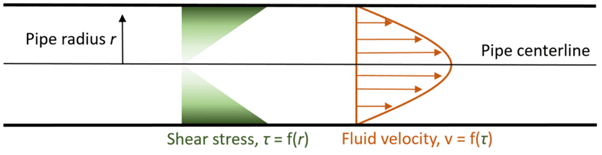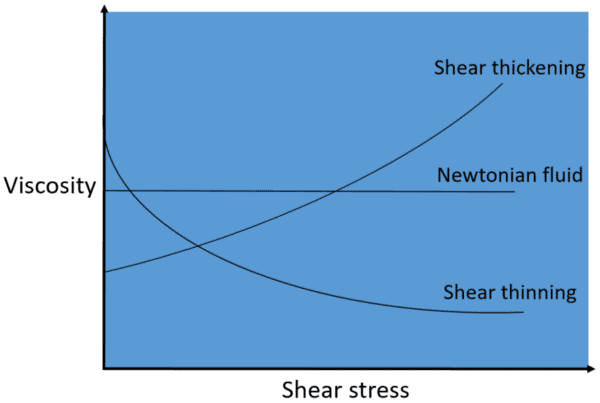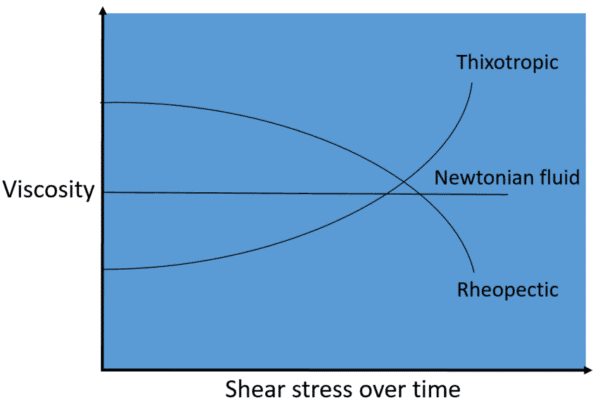Home >

# Flowing non-Newtonian fluids

Often, it may be challenging seeking out the correct tools to calculate or control non-Newtonian fluids. It is calculated that there may be up to a 20% deviation in accuracy when flow technologies such as electromagnetic meters are used for these fluids. Up to a 15% deviation is shown in Ultrasonic flow meters when used for non-Newtonian fluids  when transitioning between turbulent and laminar flow. In this blog, we will discuss the challenges posed by non-Newtonian fluids and how Coriolis flow instruments have proven to be the most successful solution.

## What are non-Newtonian fluids

An example of a Newtonian fluid that displays a constant viscosity regardless of any applied force is Water. However, depending on the type and magnitude of forces exerted on them, viscosity is changed in a non-Newtonian fluid.

 Newtonian fluids (examples) Non-Newtonian fluids (examples) Water AdBlue Alcohol Blood Glycerol Soap solutions Gasoline Honey

### The effect of shear stress

Molecules inside the fluid moving past each other cause shear forces for any process fluid moving through a pipe. Viscosity in Newtonian fluids  is not changed due to shear forces and the velocity profiles of the flows are predictable.

A decrease in velocity is caused when shear stress is increased near the pipe walls by the flow of Newtonian fluids through a pipe, as seen in the diagram below. Slower fluid flow at the pipe walls and faster fluid flow at the centre of the pipe is the result of this. The resulting velocity profile is then predictable as a function of the shear stress.Figure 1. Newtonian flow through a pipe

In the case of Non-Newtonian fluids, velocity profiles are not as predictable. This is due to the impact of the shear stress not only on the viscosity of the fluid, but also the velocity. The velocity profile through the pipe may be rendered unpredictable, changing and asymmetrical when the viscosity of the fluid changes as a function of this stress.

### How does shear stress affect viscosity for non-Newtonian fluids?

Non-Newtonian fluids can be classified into one of four groupings, characterized by the effect shear stresses have on their viscosities.

1. Dilitant (shear thickening) fluids:viscosity increases with shear stress
2. Pseudoplastic (shear thinning) fluids:viscosity decreases with shear stress
3. Thixotropic fluids:viscosity increases with shear stress over time
4. Rheopectic fluids:viscosity decreases with shear stress over timeFigure 2a. Effect of shear stress on non-Newtonian fluids (shear thickening & thinning)Figure 2b. Effect of shear stress on non-Newtonian fluids (thixotropic & rheopectic)

### What other conditions affect non-Newtonian fluids?

Further variability may be presented to a non-Newtonian fluids behaviour due to process conditions. The magnitude of shear stress that is present as well as the fluid’s viscosity and velocity profile is impacted by the changing of parameters such as pipe diameter, flow rate and pressure.

## Flowing non-Newtonian fluids using Coriolis technology

The behaviour of fluids used in the process is important to consider when selecting a flow meter or controller. There is a difference in velocity profiles and behaviour when transitioning between laminar and turbulent flows when comparing non-Newtonian and Newtonian fluids. These characteristics decrease the measurement accuracy of flow meters that rely on expected flow velocity profiles.

Coriolis mass flow instruments are able to accurately measure and control non-Newtonian fluid flow rates due to their unique operating principle which uses inertial forces to directly determine mass flow rates. This was validated by a University of Liverpool study that found Coriolis flow devices to operate within the specified accuracy tolerance when used with non-Newtonian fluids.

Awareness of the technologies limitations is important despite the fact that Coriolis instruments have been shown to be the most impactful solution for flowing non-Newtonian fluids. There a select amount of non-Newtonian fluids that may not be compatible with certain Coriolis devices and are also highly viscous, some examples are cement slurries, Yoghurt and Honey.

Less viscous non-Newtonian fluids, such as polymer solutions, are perfect for use with Coriolis instruments like Lab Unlimited’s CODA-Series mass flow meters and controllers. For example, the substance AdBlue is a non-Newtonian fluid solution mixture of 32.5% urea and 67.5% DI water. This liquid is also known as a diesel exhaust fluid, and is poured into many diesel truck engines so it can reduce emissions by breaking down soot and unburnt fuel into nitrogen and water byproducts. Testing of AdBlue efficacy and usage often requires accurate measurement of flow over set time periods. The accuracy of standard flow measurement technologies is hindered by the non-Newtonian fluid properties. CODA is an excellent choice for this solution as it is able to flow non-Newtonian fluids, and has the added benefit of a totalizer function.

 To Find Out More

Copyright 2013 Lab Unlimited Sitemap | Terms & Conditions | ! Product images are for illustrative purposes only !